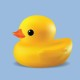# Chocolate Arrays

Lately, I’ve been seeing arrays everywhere I go: at the grocery store, at the pharmacy, at the farmers’ market. And, of course, at Costco. The big, bad bulk retailer is bursting with interesting items arranged in perfect columns and rows.

Naturally, I made a beeline for the chocolate.

What follows is a quick-image string for exploring the associative property, the patterns that occur when multiplying, and the relationship between columns and rows. It supports the development of some key strategies for multiplying: doubling and halving to maintain equivalence, doubling a dimension to double to product, and using partial products to solve.

Show the images for a few seconds each, one at a time, to your students. I have provided some question prompts if you’re interested, and my rationale for choosing each image appears below.

Have fun!

Look at the chocolates in the picture. How many do you see? How did you see them?Record an expression or equation if it gets mentioned: “three by two” or “three rows with two chocolates in each row” can be expressed as 3 x 2 = 6

How many chocolates are in this picture? How do you know?Record any expressions or equations that come up: “Three rows of four” or 3 x 4 = 12. Pay close attention to what students notice about this array. There will likely be some discussion of doubling. Where is the doubling in this picture? What gets doubled?

What about now? How many chocolates do you see?
How does this picture compare to the picture before?
Do you think you can predict how many chocolates are in a whole box?This image clearly shows another “double.” It’s important to discuss which dimension doubles, and which one doesn’t, and what happens to the total number of chocolates when this doubling occurs. Nudge for any generalizations that might be made about this.

Now how many do you see? How is this picture the one the same as the previous one and how is it different?Here is another array of 24, but the number of rows has doubled while the length of each row has been cut in half. Listen closely to students’ descriptions of this array as compared to the previous one. Record equations as they come up.

How many now?
How many chocolates are in the whole box?There are lots of ways to see this one!

There are eight “copies” of the first image: 8 (3 x 2),

Or four of the second image: 4 (3 x 4),

Or two of the third and fourth images: 2 (3 x 8) and 2 (6 x 8), respectively.

Can students find the previous arrays in this picture?

What happened here? How many chocolates?This reinforces the idea that doubling one dimension doubles the product.

There are different ways to describe this; students might simply see two 6 x 8 arrays: 2 (6 x 8) or 6 x 8 + 6 x 8.

They may also see 6 rows of 16, or 6 x 16. Discuss how these different ways of seeing this array are related.This one shows the 6 x 8 array doubled in a different way. For students who are beginning to explore volume, the added dimension is extremely important. This might prompt a rich discussion about layers. Here we see (6 x 8) x 2.

1.shouldbequiltin says:
2.Bee Gee says:
3.Kelly A. Serrano says: